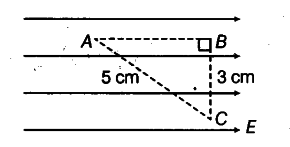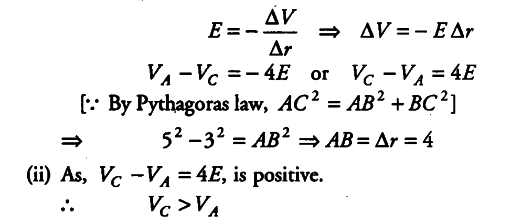# A test charge q is moved without acceleration

A test charge q is moved without acceleration from A to C along the path from A to B and then from B to C in electric field E as shown in the figure.(i) Calculate the potential difference between A andC.
(ii) At which point (of the two) is the electric potential more and why?

(i) Electric field intensity and potential difference are related as,Potential is greater at point C than at point A, as potential decreases along the direction of electric field.